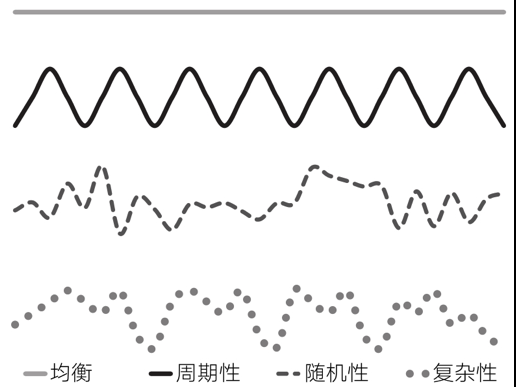## 信息熵

$$H(p_1, p_2, p_3, p_4 … P_N) = - \sum ^N_{i=1} p_i log_2(p_i)$$

## 熵的应用和最大熵

• 最大化：对于所有的N，当$p_i = 1/N$ 时熵最大
• 零性：$H(1,0,0 …0) = 0$
• 可分解性：如果一个事件是由两个独立事件共同发生造成的那个$H(P) = H(P1) + H(P2)$• 均匀分布：给定范围［a，b］，使熵最大化。
• 指数分布：给定均值$\mu$，使熵最大化。
• 正态分布：给定均值$\mu$和方差$\sigma^2$，使熵最大化。